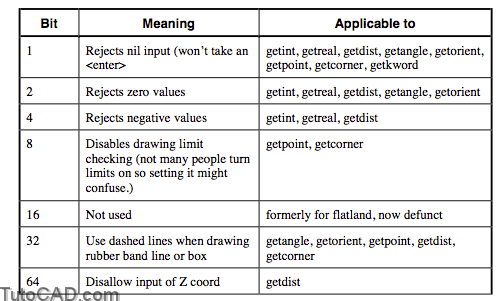# Restricting Acceptable Input Values with autolisp in AutoCAD

One of the most tedious tasks for the AutoLISP programmer is error trapping, making sure the routine can handle all varied inputs from the user. By restricting the acceptable input values with the (initget) function, you can control information supplied by the user.

Explanation: the (initget) function

(initget [bits])

The bits is an integer that refers to the following restrictions to input:• The (initget) function affects only the next (getxxx) function that is encountered.
• None of the initget bits affect the (getstring) function; we will learn how to error-trap string input later in this chapter.
• More than one of these restrictions can apply simultaneously by summing the corresponding integers. It is a good idea to show the addition in the routine rather than the sum. This way someone else reading the routine will know what is going on.

(initget (+ 1 2 4))

Examples

Command: (initget 1)
nil

Command: (setq DST (getdist “Enter distance: ”))
Enter distance: <enter>
Requires numeric distance or two points.

Try again: 3.2
3.2

(initget (+ 1 2 ))
nil
Command: (setq SF (getreal “Enter scale factor: ”))
Enter scale factor: <enter>
Requires numeric value.

Try again:
Value must be nonzero.

Try again: 2
2.0

PRACTICE

1. Write out the initget/getxxxx combination that will be appropriate for specifying “Number of rows:”

2. Add the appropriate (initget) functions to the BOX1.LSP file.

(defun c:BOX1 ()
(graphscr)
(setq CE-SAV (getvar “cmdecho”))
(setvar “cmdecho” 0)
(initget 1)
(setq PT1 (getpoint “\nFirst corner of box: ”))
(initget 1)
(setq PT2 (getcorner PT1 “\nOpposite corner: ”))
(setq x1 (car PT1))
(setq x2 (car PT2))